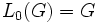# Varying nilpotence

This is a survey article describing variations on the following: nilpotent group
View other variational survey articles | View other survey articles about nilpotent group

## Introduction

Nilpotence is one of the most pivotal group properties. Although it originated from the theory of nilpotent Lie algebras, nilpotence is now a fairly important notion in group theory. Several variations of this property have been studied. There are, broadly, four kinds of variation:

• Variations coming from the central series definition
• Variations that are indistinguishable if we are working only with finite groups. In other words, we write down a long list of equivalent definitions of finite nilpotent group, and generalize each of these in a different way to infinite groups
• Variations that try to remedy the gap between nilpotence and Abelianness: In other words, these are group properties that (at least for finite groups) live somewhere between nilpotent and Abelian
• Variations that try to remedy the gap between nilpotence and solvability: These are group property that (at least for finite groups) live somewhere between nilpotent and solvable

## Variation in terms of the lower central series

Further information: Lower central series

A group is nilpotent if its lower central series terminates at the identity element in finitely many steps. The lower central series$L_c(G)$ is defined, for finite$c$, as follows:$L_0(G) = G$ and$L_{i+1}(G) = [G,L_i(G)]$.

Now, one way we could extend this notion is by extending the lower central series to all ordinals. For any ordinal$\alpha$, define$L_\alpha(G) = \bigcap_{\gamma < \alpha} L_\gamma(G)$ if$\alpha$ is a limit ordinal, and$L_{\alpha + 1}(G) = [G,L_\alpha(G)]$.

This gives us a transfinite lower central series, and is the subject of the coming definitions. Note that the transfinite lower central series must terminate at some ordinal, because the group is a set.

### Residually nilpotent group

Further information: residually nilpotent group

A residually nilpotent group is a group for which$L_\omega(G)$ (in the above language) is the trivial subgroup, where$\omega$ is the first infinite ordinal. In other words, the intersection of all the finite terms of the lower central series is trivial. Another way of saying this is that every non-identity element avoids some member of the lower central series, or equivalently, that for every non-identity element, there is a normal subgroup not containing it, with a nilpotent quotient group.

Residual nilpotence is very different from nilpotence. For instance, free groups are residually nilpotent, but are very far from nilpotent. In fact, free groups are centerless, so if we were to look at the upper central series, they'd be as far from nilpotent as possible. Further information: free implies residually nilpotent

Any subgroup of a residually nilpotent group is residually nilpotent, but a quotient of a residually nilpotent group ned not be residually nilpotent. The key obstacle is the fact that taking an intersection does not descend well to the quotient (the quotient of an intersection could be much smaller than the intersection of the quotients).

### Hypocentral group

Further information: hypocentral group

A hypocentral group is a group where the lower central series eventually terminates at the identity. This could happen at any ordinal. Any residually nilpotent group is hypocentral, but the property of being hypocentral is substantially weaker.

Any subgroup of a hypocentral group is hypocentral, but a quotient of a hypocentral group need not be hypocentral. That's because taking quotients doesn't behave well with intersections.

For an arbitrary group, the subgroup at which the lower centra lseries eventually stabilizes is termed its hypocenter.

## Variation in terms of the upper central series

Further information: Upper central series

A group is nilpotent if its upper central series terminates, in finitely many steps, at the whole group. We define the upper central series as follows.$Z^0(G)$ is trivial, and$Z^i(G)$ is a subgroup containing$Z^{i-1}(G)$ such that$Z(G/Z^i(G)) = Z^{i+1}(G)/Z^i(G)$.

As for the lower central series, we can define a transfinite upper central series. Here, for limit ordinals, we use unions. The unions are still subgroups since they are unions of ascending chains of subgroups.

### Hypercentral group

Further information: hypercentral group

A hypercentral group is a group where the upper central series eventually stabilizes at the whole group. For a general group, the subgroup at which the upper central series stabilizes is termed the hypercenter, so a hypercentral group is a grou pwhere the hypercenter equals the whole group.

Hypercentral groups behave fairly differently from hypocentral groups.

## The finite groups route to variation

The following are characterizations for a finite group to be a finite nilpotent group:

We can use these alternative characterizations and generalize to the infinite group situation.

### Groups with the normalizer condition

Further information: normalizer condition, nilpotent implies normalizer condition, normalizer condition not implies nilpotent

A group is said to have the normalizer condition if it satisfies the following equivalent conditions:

A hypernormalized subgroup is a subgroup whose hypernormalizer is the whole group. The hypernormalizer is the subgroup where the transfinite ascending chain obtained by taking normalizers, stabilizes.

The normalizer condition is fairly strong, but is strictly weaker than nilpotence. In other words, there are subgroups satisfying the normalizer condition, that are not nilpotent.

### Other variations

Other variations include notions like:

## Remedying the gap between nilpotence and Abelianness

### Group generated by Abelian normal subgroups

Further information: Group generated by Abelian normal subgroups

### ACIC-group

Further information: ACIC-group

## Remedying the gap between nilpotence and solvability

### Supersolvable group

Further information: supersolvable group